# How to read the Confusion Matrix

The Confusion Matrix is a square table representing the predictions of a classification model. As indicated by its name, the table shows how the model is confused when predicting. By examining the Confusion Matrix, we can derive many evaluation measures (like accuracy, recall, precision, etc.)

While there can be many classes in a Classification problem, the case with binary-label is the most common and simple. To illustrate, let’s suppose we have 140 binary-labeled samples in our dataset and the resulting prediction taken from the predictive model is:

To summarize the table: there are 50 positive samples, among which 30 are predicted correctly while the other 20 are mistakenly predicted to be negative. There are also 90 negative samples, 50 of them are indeed predicted as negative while the remaining 40 are erroneously classified as positive.

To make it more concrete, each cell of the table is assigned a name, those are True Positive, True Negative, False Positive and False Negative.

To not get confused with these names, remember that: Because the Confusion Matrix is created to evaluate how a model works, each cell’s name is all about the prediction of the model. For example, False Positive (FP) is the number of predictions that say the labels are positive but those predictions are false. Many people are dazed with these names, e.g. sometimes mistakenly thinking that the Positive term is about the actual label of the data, so, be careful.

The confusion matrix gives us insights into how the model works. For example, looking at the matrix and do some little computation, we get that there are more samples to be predicted accurately (80) than wrongly (60).

To be more formal, let’s take a look at some of the most frequently used measurements as shown in the table below:

The first and most popular one should be the Accuracy of the model, which implies the percentage of true predictions overall.

Accuracy =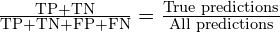In the above example,

Accuracy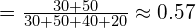The Precision expresses how precise the model is when it spots a positive case.

Precision =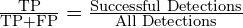Plug in the above example,

Precision =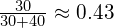The True Positive Rate (TPR), or Recall, is the proportion of the True Positive count over the maximum number of possible True Positives we can have with any models.

TPR =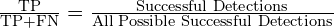For the example,

TPR =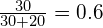The False Positive Rate (FPR), similarly, is the proportion of False Positive count over the maximum number of possible False Positive we can have with any models.

FPR =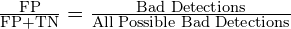This gives,

FPR =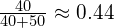Even though we only examined the matrix in a binary classification basis, in practice, confusion matrices can also represent the predictions of multi-class classification, such a matrix will have more than 2 rows and 2 columns. However, be reminded that in those cases, each measurement described above should be calculated for each category separately. For example, in a 3-class problem, we have to calculate True-Positive-class-1, True-Positive-class-2, and True-Positive-class-3 instead of just True Positive.

Conclusion

In this blog post, we get to know how to read the confusion matrix and make familiar with some typical measurements that are computed from a confusion matrix.

Apart from the mentioned measurements, there are also many other metrics that can be derived from confusion matrices, for instance, F1-score, Area under the ROC Curve and Area under the Precision-Recall curve. To choose to use which one for a problem depends upon the problem itself.

References: